## Saturday, February 01, 2020

### [ozjcrzwx] Ulam spirals

Here is the classic Ulam spiral of primes with 1 as the center number, 2 to its right, spiraling counterclockwise.  Image is 320x320 (so numbers up to 320^2 = 102400), magnified by 2.  There are primepi(320^2) = 9805 primes in this image.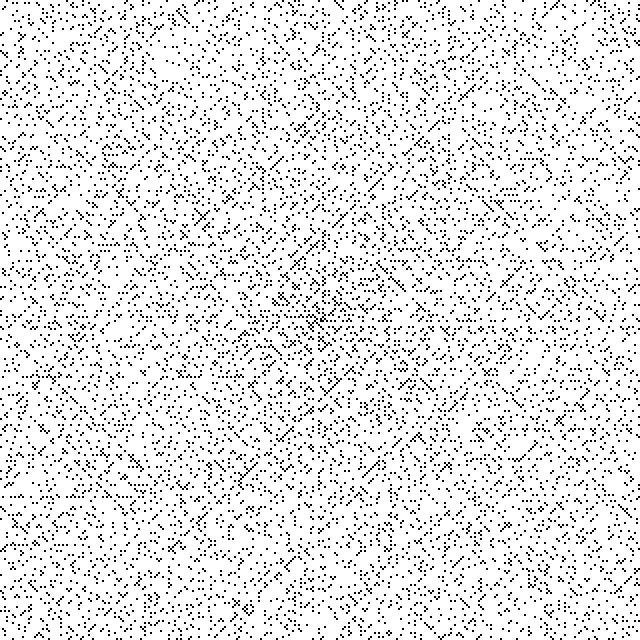1920x1920, reduced in HTML by 1/2.  Right click and View Image to view original size.  This covers numbers up to 1920^2 = 3686400.  There are primepi(1920^2) = 262479 primes (black pixels) in this image.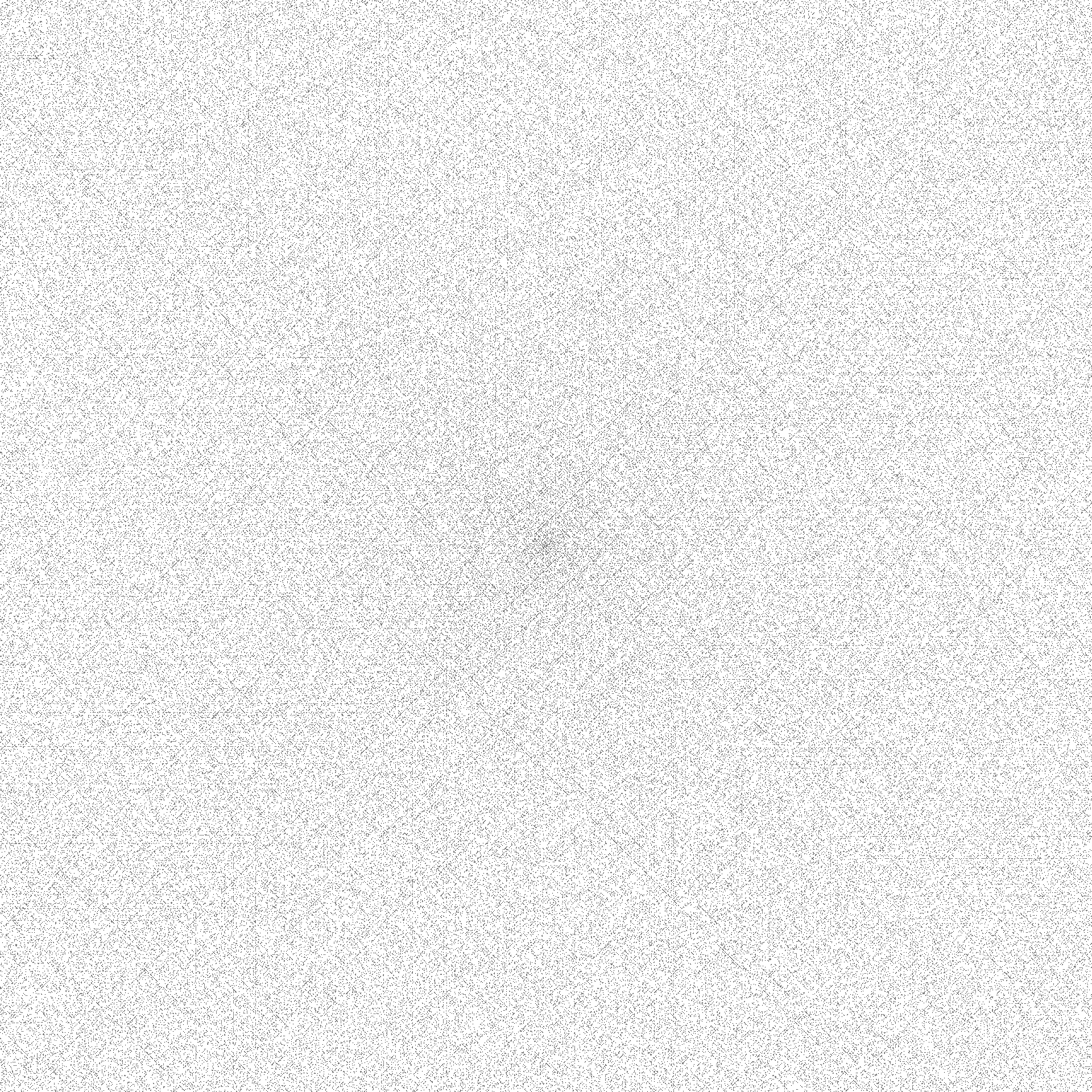Here is the Ulam spiral starting with 41 as the center number, with its famously prominent long diagonal line of primes through the origin.  That diagonal seems to remain rich in primes beyond its unbroken streak near the origin (Hardy and Littlewood's Conjecture F).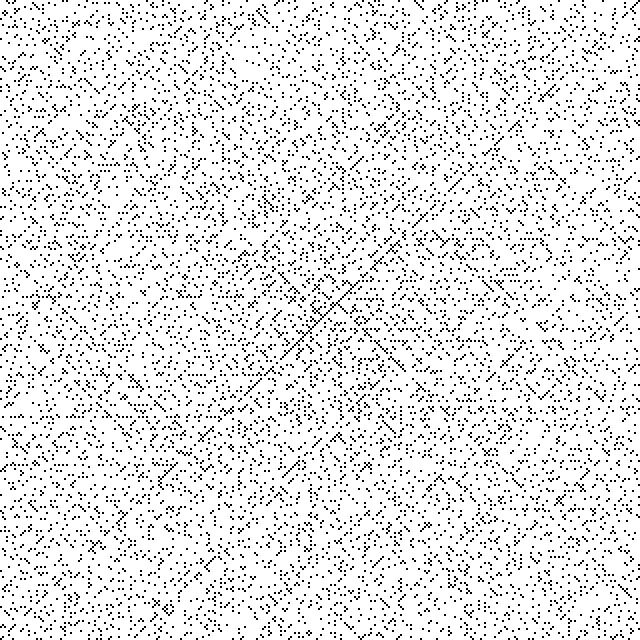Instead of putting consecutive natural numbers along the spiral, we consider putting the numbers of arithmetic progressions a*n + b (n = 0, 1,...).

Here is an Ulam spiral of the odd numbers 2n+1.  It looks very different, with horizontal and vertical lines seemingly dominating diagonal lines.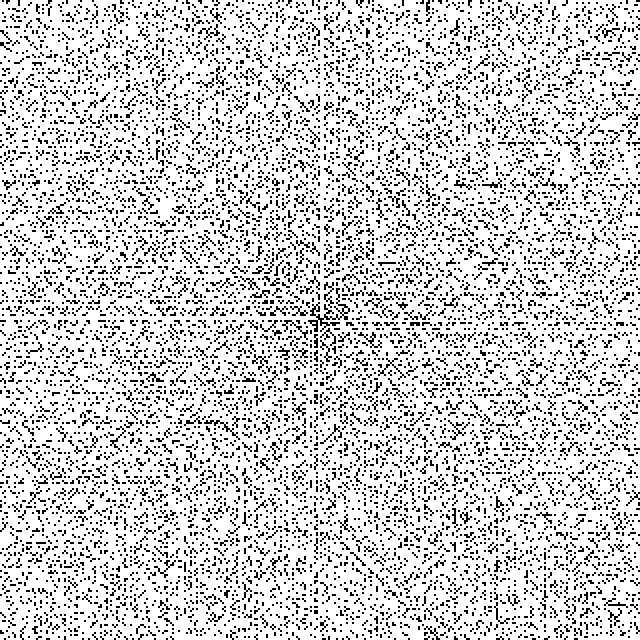The corresponding Ulam spiral of the even numbers would have one dot for the prime 2 and no other dots.

Here are Ulam spirals marking the primes among numbers of the form 3n+1 and 3n+2.  When the multiplier is odd (including the original Ulam spiral with multiplier 1), it is impossible for orthogonally adjacent pixels to both be black (except near the origin).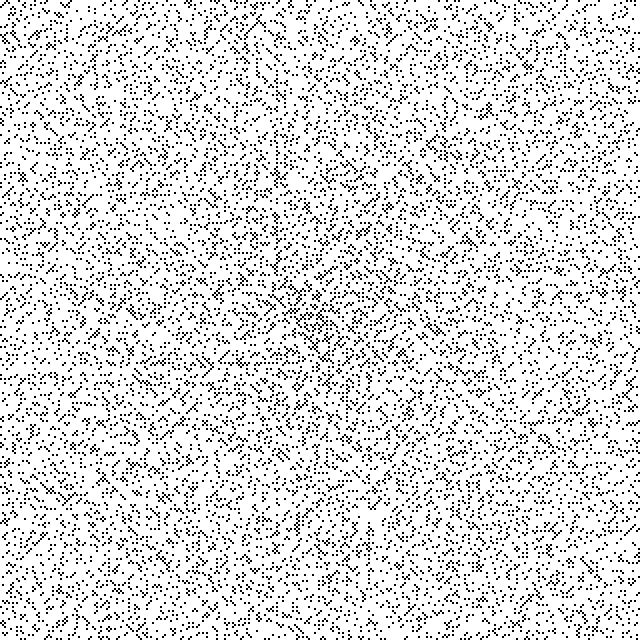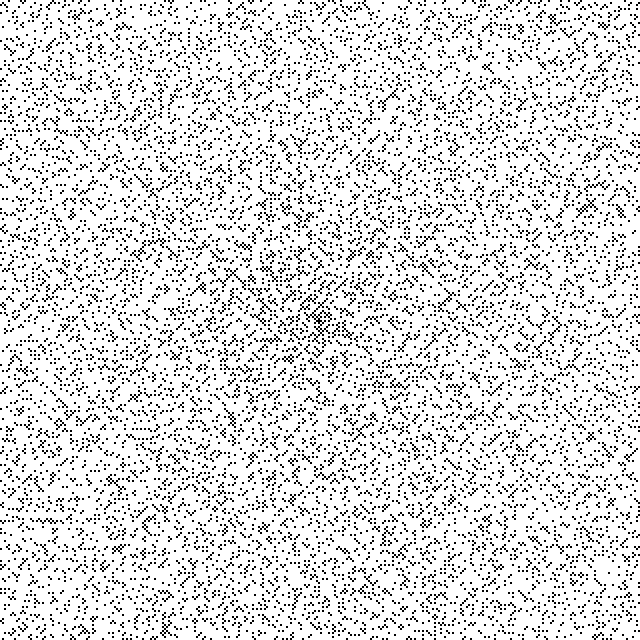4n+1 and 4n+3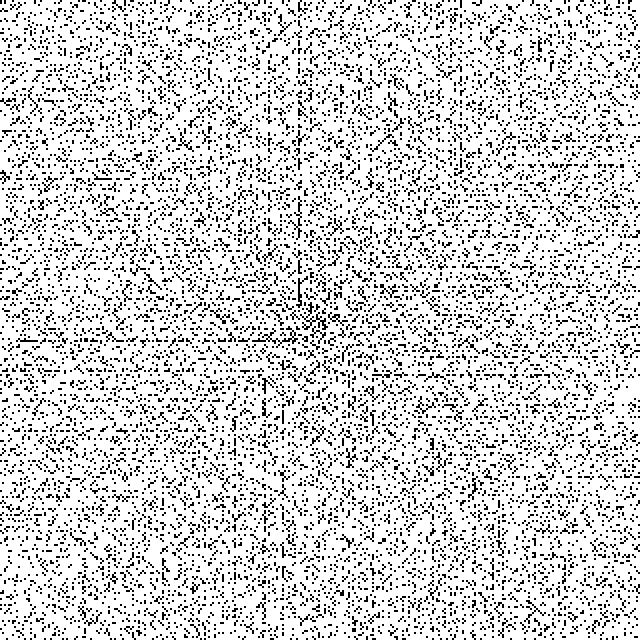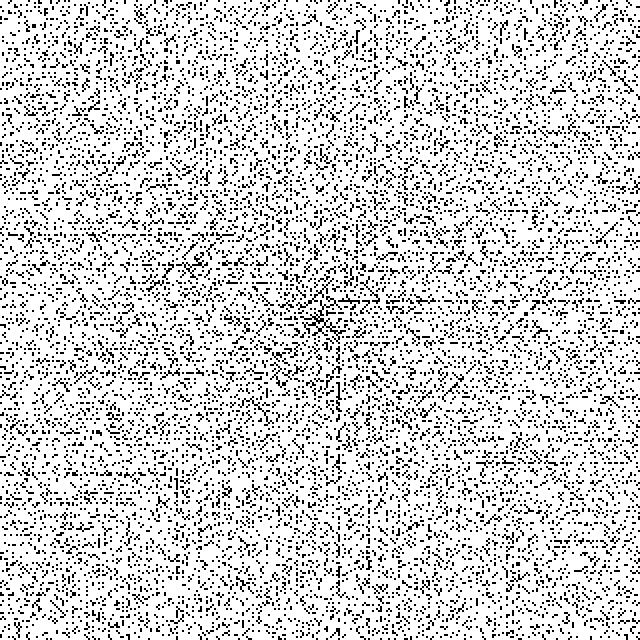5n+1, 5n+2, 5n+3, and 5n+4.  The offset b does not affect the character of the image very much.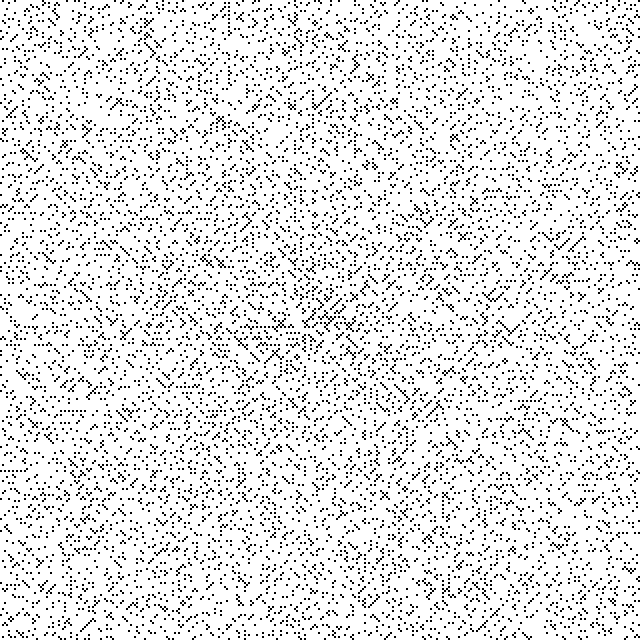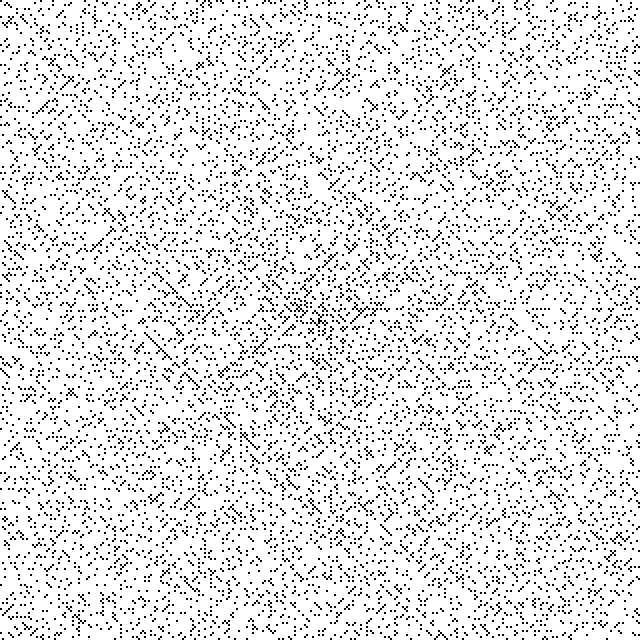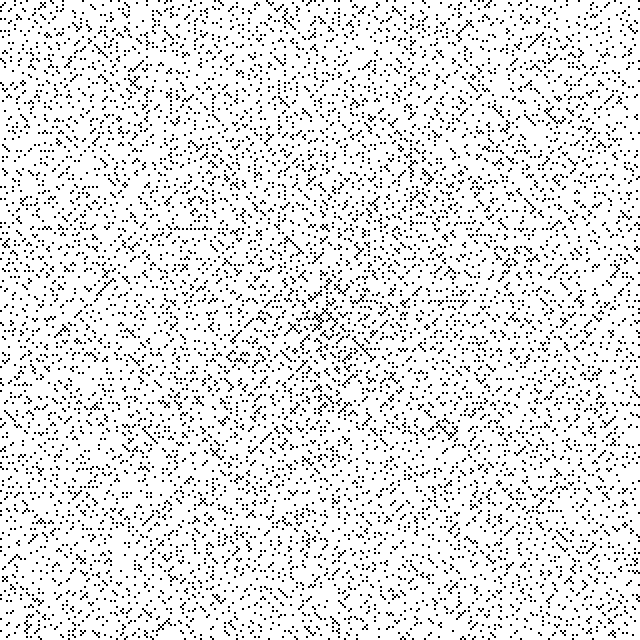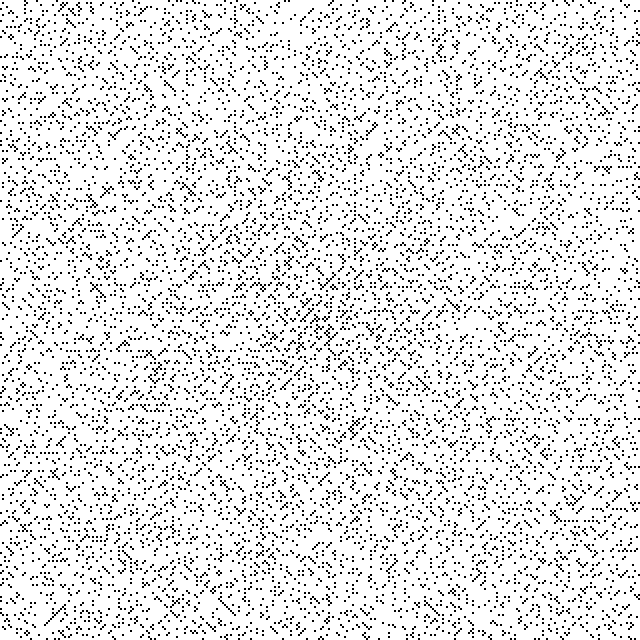6n+1 and 6n+5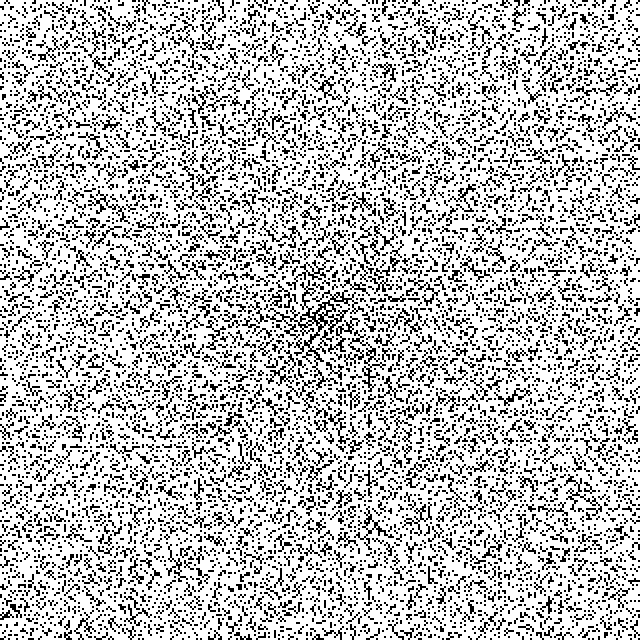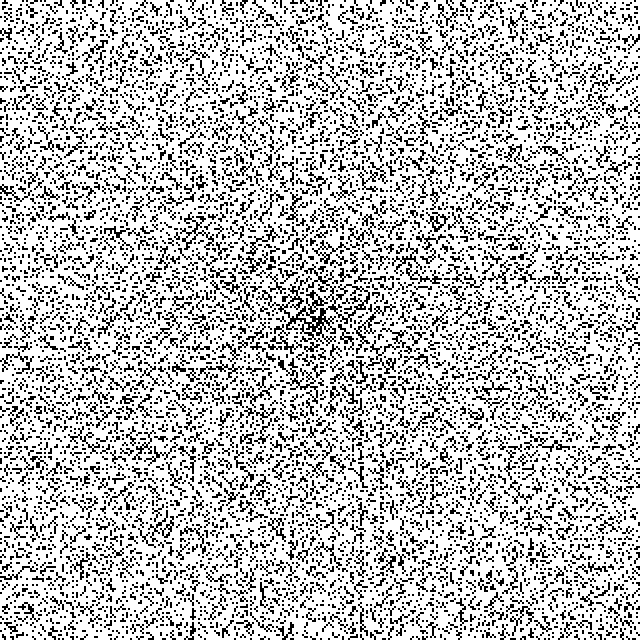7n+1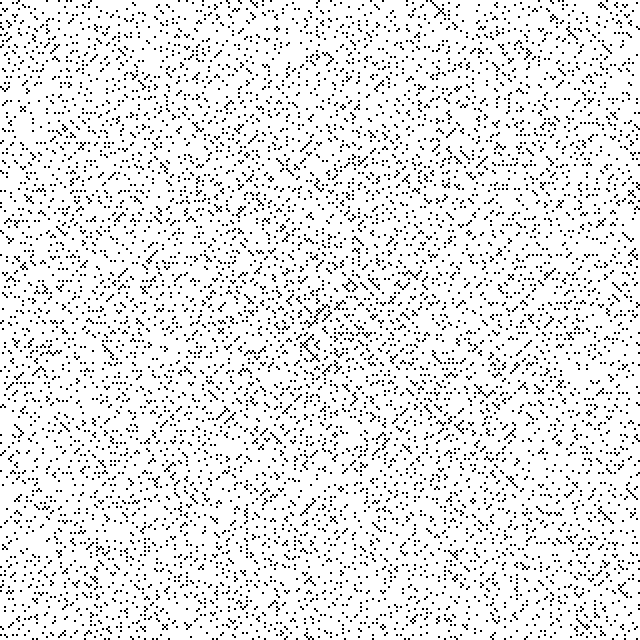8n+1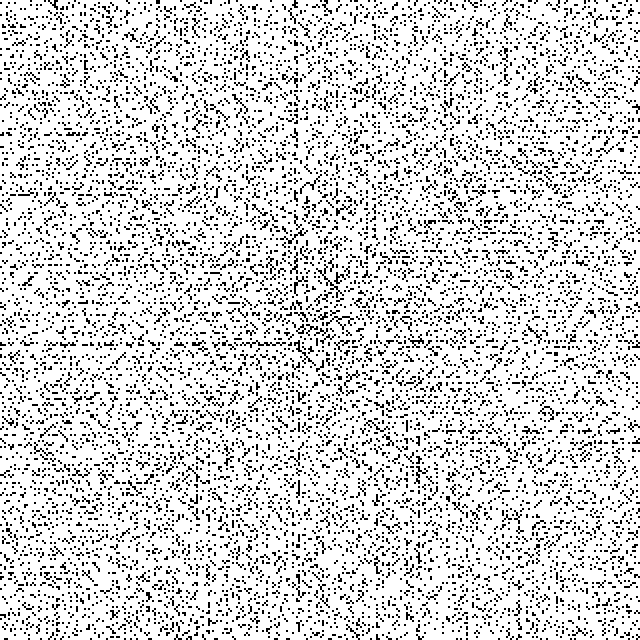9n+1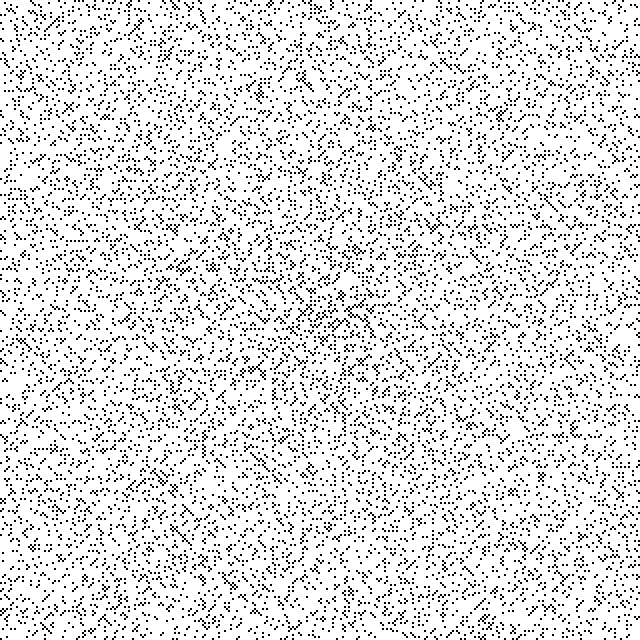10n+1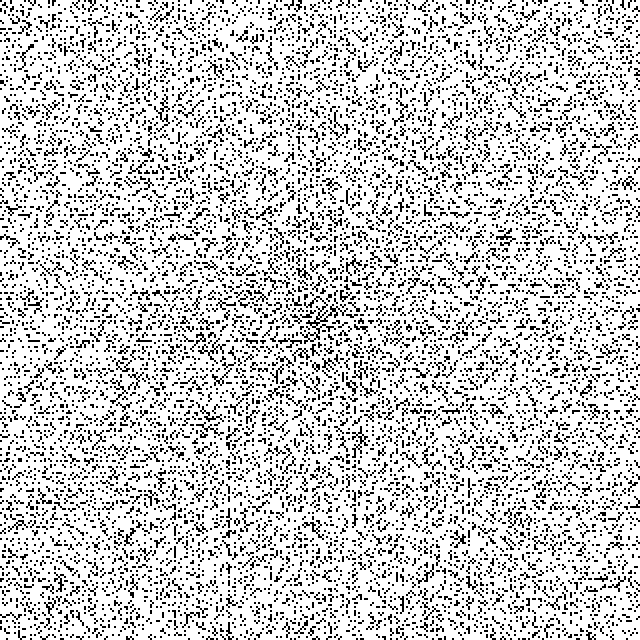11n+1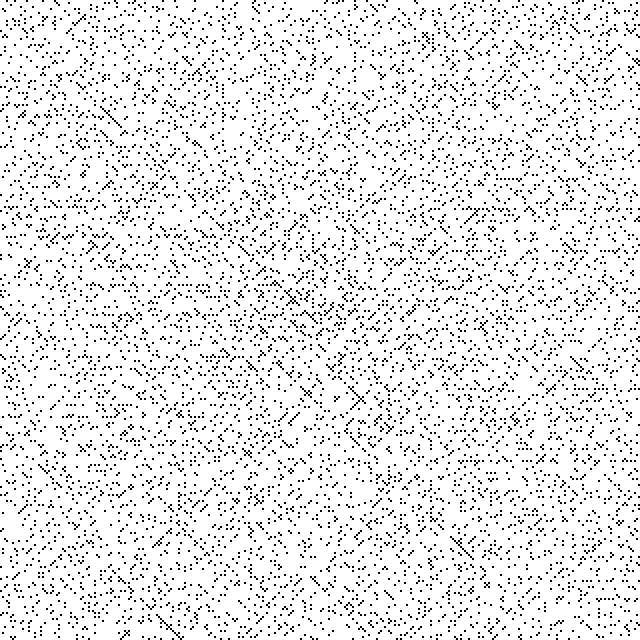We conjecture that the way the image looks strongly depends on the factorization of the multiplier a, whether it has many small prime factors.  Here is 15n+1.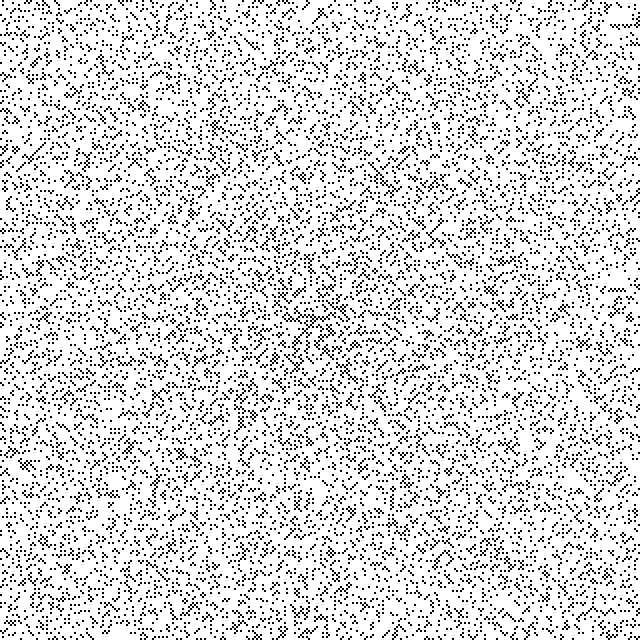Finally, we briefly examine a different type of spiral, one that does not densely fill the plane, but leaves gaps (in gray) between turns.  This spiral is a little bit more regular than the normal Ulam spiral.  The length of each straight segment grows by 1 every quarter turn.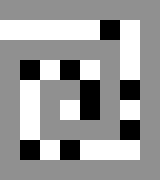Bigger version.  This spiral is called `l2alternate` in the source code.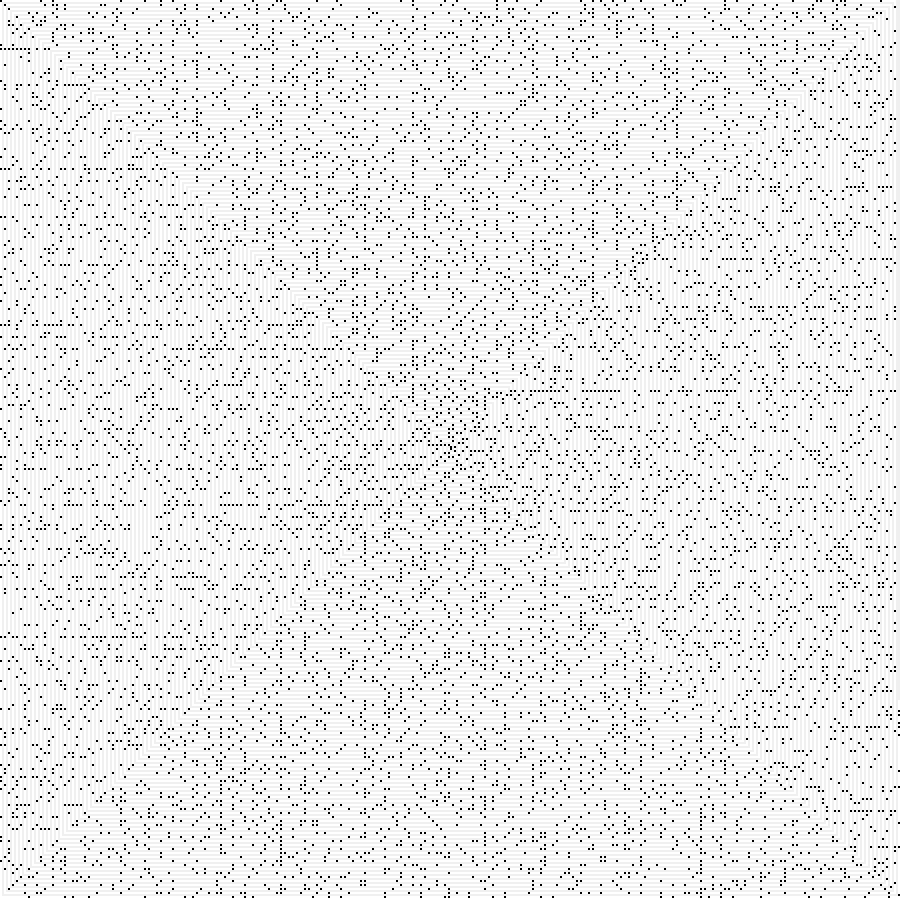Here is the Haskell program used to generate these images.  Command line arguments are width, a, and b, for example

./ulam-spiral 320 5 3 > output.png

to generate the 320x320 5n+3 image above.

Some notes on implementation:

To calculate the coordinates of pixels in the classic Ulam spiral, observe that the spiral is composed of a sequence of L-shaped segments of smoothly growing size: 1-2-3, 3-4-5-6-7, 7..13, 13..21, etc.(Image source)

First, we consider drawing one L-shaped segment of a given size.  The following function emits a list of delta vectors, how to get from one pixel in the L to the next pixel to be drawn.  (This portion somewhat resembles the LOGO programming language.)  The argument n is the size of the L, and dir (a unit vector) is the initial direction we are facing, i.e., the initial delta vector.

``` > type Pair = (Integer,Integer); > -- one L of a spiral > l1 :: Integer -> Pair -> [Pair]; > l1 n dir = List.genericReplicate n dir ++ List.genericReplicate n (rotate90 dir); > -- counterclockwise > rotate90 :: Pair -> Pair; > rotate90 (x,y) = (negate y,x); ```

```*Main> l1 3 (1,0)[(1,0),(1,0),(1,0),(0,1),(0,1),(0,1)] ```

This corresponds to the L from 7 through 13 in the picture above.

We elide a few straightforward steps of generating and concatenating all the delta vectors of the infinite sequence of Ls growing in size.  After that, all the coordinates can be generated from the delta vectors with `scanl`, starting at (0,0).  The output is an infinite list of coordinates, from which we will take as many as we need for a desired image size.

``` > allcoords :: [Pair]; > allcoords = scanl addcoords (0,0) spiralsteps; *Main> take 9 allcoords [(0,0),(1,0),(1,1),(0,1),(-1,1),(-1,0),(-1,-1),(0,-1),(1,-1)]```

Here is how to add coordinates in point-free style:

` > addcoords :: Pair -> Pair -> Pair; > -- addcoords (a,b) (x,y) = (a+x, b+y); > addcoords = Data.Biapplicative.biliftA2 (+) (+); `

Is there a combinator f with which addcoords could have been defined "f (+)", i.e., use the same binary operator on both components?  It seems like a combinator someone may have already written and packaged.

We use the JuicyPixels package to provide a raster onto which we plot pixels.  We use MutableImage in the IO monad.  Here is the call to newMutableImage with a pedagogical type annotation for its return type:

``` > {-# LANGUAGE ScopedTypeVariables #-} > import Control.Monad.Primitive(PrimState);> import qualified Codec.Picture.Types as JuicyT;> import qualified Codec.Picture as Juicy; ... > do { >  image :: JuicyT.MutableImage (PrimState IO) Juicy.Pixel8 <- JuicyT.newMutableImage size size; > } ```

The "PrimState IO" type argument is a bit arcane.  PrimState is an "associated type", or "associated type family", or "type synonym family".  This is a feature within the broader Type Families GHC feature.  If we replace (PrimState IO) with _ , the Partial Type Signatures GHC feature infers that GHC.Prim.RealWorld should go in that hole, but that seemed a bit too bare metal, so via inspired guesswork, we found PrimState IO instead.

(The current version of the code uses createMutableImage, which has the same return type as newMutableImage.)

The code has a space leak.  Memory usage is 637 bytes per pixel for image sizes around 2000x2000, and the overhead per pixel gets worse for larger images, so we cannot generate images much larger than that.  Someday, we may put some effort into figuring out what is going wrong.

A previous use of Juicypixels, running into a space leak then as well.